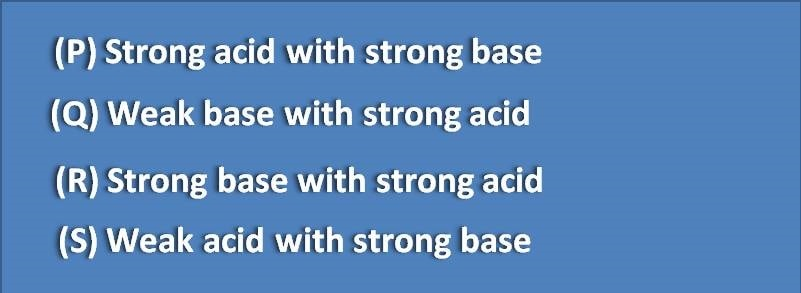1. Home
2. â€º
3. Test papers
4. â€º

# MCQ on Principles of titrimetric analysis: Page-5

Calculate the pH of solution of a weakly acidic salt at the following concentration.(A) 3

(B) 11

(C) 5.5

(D) 13

Which of the following substance achieves equilibrium when dissolved in solution

(A) HCl

(B) Acetic acid

(C) NaOH

(D) Sulphuric acid

Which of the following are acidimetric titrations(A) Q,R

(B) P,S

(C) Q,S

(D) P,R

Systematic errors are due to

(A) Analytical method

(B) Equipment

(C) Analyst

(D) All of the above

All of the following are the ideal requirements of a primary standard, except

(A) Not hygroscopic

(B) Low molecular mass

(C) Known impurities